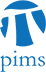## SCAIM Seminar: Michael Overton (NYU)

• Date: 11/09/2010
Lecturer(s):
Michael Overton, Computer Science, Courant Inst. NYU, New York
Location:

University of British Columbia

Topic:

Characterization and Construction of the Nearest Defective Matrix via Coalescence of Pseudospectral Components

Description:

Let $A$ be an $n\times n$ matrix with $n$ distinct eigenvalues. Its open $\epsilon$-pseudospectrum is the set of points in the complex plane which are eigenvalues of a matrix $B$ with $||A-B|| < \epsilon$. For sufficiently small $\epsilon$ the pseudospectrum consists of $n$ disjoint connected components (one around each eigenvalue), and as $\epsilon$ is increased these components coalesce with one another. Let $c(A)$ be the supremum of all $\epsilon$ for which the pseudospectrum has $n$ components.

Let $w(A)$ be the distance from $A$ to the set of defective matrices, using either the 2-norm or the Frobenius norm. Demmel and Wilkinson independently observed in the early 1980s that $w(A)\geq c(A)$, and equality was established for the 2-norm by Alam and Bora in 2005. We give new results on the geometry of the pseudospectrum near points where coalescence of the components occurs, characterizing such points as the lowest generalized saddle point of the smallest singular value of $A-zI$ over $z\in \C$. One consequence is that $w(A) = c(A)$ for the Frobenius norm too, and another is the perhaps surprising result that the minimal distance is attained by a defective matrix in all cases. Our results suggest a new computational approach to approximating the nearest defective matrix.

Schedule:

12:30pm -- 2:00pm, WMAX 110

Pizza and pop will be provided!

Other Information:

For details, please visit the official website at